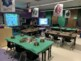# Multiplication Bootcamp Room Transformation 3rd, Eureka Math, Module 3, Lesson 8Subject
Resource Type
File Type

Presentation (Powerpoint) File

(98 MB|13 pages)
Product Rating
Standards
• Product Description
• StandardsNEW

Multiplication Bootcamp Room Transformation 3rd, Eureka Math, Module 3, Lesson 8

-This PowerPoint aligns with 3rd Grade Eureka Math Module 3, Lesson 8. When you have the cover slide in presentation mode fun music will play in the background to get students pumped up for the lesson.

*YOU GET*

-Example Videos & Photos for Inspiration

PPT includes slides for:

-Learning Target

-Skip Counting (Fun way to practice fluency. Students skip count to the Cha Cha Slide)

-Pattern sheet and adding 6 & 7 mentally

-Application problem (Also with fun music playing in the background when PPT is in presentation mode)

-Concept Development

-Problem Set

It would mean so much to me if you would leave feedback..even one or two words! Thanks so much!!! :)

Solve two-step word problems using the four operations. Represent these problems using equations with a letter standing for the unknown quantity. Assess the reasonableness of answers using mental computation and estimation strategies including rounding.
Fluently multiply and divide within 100, using strategies such as the relationship between multiplication and division (e.g., knowing that 8 × 5 = 40, one knows 40 ÷ 5 = 8) or properties of operations. By the end of Grade 3, know from memory all products of two one-digit numbers.
Understand division as an unknown-factor problem. For example, find 32 ÷ 8 by finding the number that makes 32 when multiplied by 8.
Apply properties of operations as strategies to multiply and divide. Examples: If 6 × 4 = 24 is known, then 4 × 6 = 24 is also known. (Commutative property of multiplication.) 3 × 5 × 2 can be found by 3 × 5 = 15, then 15 × 2 = 30, or by 5 × 2 = 10, then 3 × 10 = 30. (Associative property of multiplication.) Knowing that 8 × 5 = 40 and 8 × 2 = 16, one can find 8 × 7 as 8 × (5 + 2) = (8 × 5) + (8 × 2) = 40 + 16 = 56. (Distributive property.)
Determine the unknown whole number in a multiplication or division equation relating three whole numbers. For example, determine the unknown number that makes the equation true in each of the equations 8 × ? = 48, 5 = __ ÷ 3, 6 × 6 = ?.
Total Pages
13 pages
N/A
Teaching Duration
N/A
Report this Resource to TpT
Reported resources will be reviewed by our team. Report this resource to let us know if this resource violates TpT’s content guidelines.